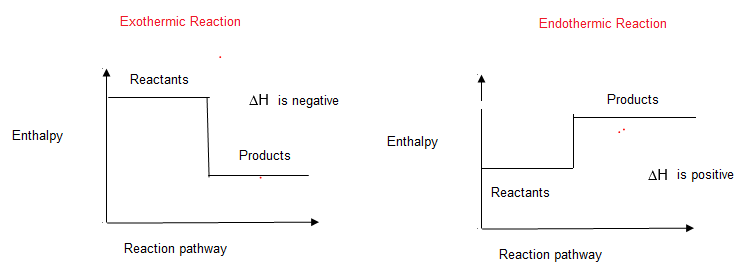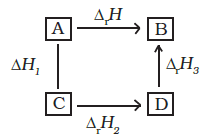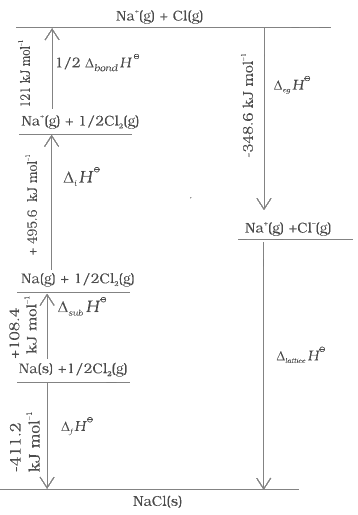# Enthalpy , Change in enthalpy in reaction And Hess's Law

## ENTHALPY (H)

Enthalpy is defined as total heat content of a system and is equal to the sum of internal energy and pressure volume work.
Mathematically
H = U + W
H = U + PV
It is state function
At constant Pressure, we know of First law of Thermodynamics
ΔU= qp +W
Or
qp = ΔU – W = ΔU + P(V2 – V1) = U2 - U1 +   PV2 -PV1
= (U2 + PV2) – (U1 + PV1 ) = ΔH
So, Change is Enthalpy is the heat absorbed or evolved by the system at constant pressure.
ΔH = +ve    (Endothermic reaction)
ΔH = -ve      (Exothermic process)
Relation between ΔH and ΔU
H1 = U1 + PV1
H2 = U2 + PV2
H2 – H1 = U2 + PV2 – (U1 + PV1)
ΔH = U2 – U1 + PV2 – PV1
=U2 – U1 + P(V2 – V1)
= ΔU + PΔV
Now Let us consider a reaction involving gases. If V1 is the total volume of the gaseous reactants, V2 is the total volume of the gaseous products, n1 is the number of moles of gaseous reactants and n2 is the number of moles of gaseous products, all at constant pressure and temperature, then using the ideal gas law, we
write,
pV1 = n1RT
pV2 = n2RT
pΔV =Δn RT
So, Enthalpy of reaction can be written as
ΔH =ΔU + ΔngRT
Heat Capacity
It is the increase in temperature (1 degree) of any substance.
So,         $C =\frac {Q}{\Delta T}$
Molar Heat Capacity
It is the heat capacity for 1 mole of a substance
$C =\frac {Q}{n\Delta T}$
Specific Heat Capacity
It is defined as the heat required to raise the temperature of unit mass of a substance by 1 degree or Kelvin.
$C =\frac {Q}{m\Delta T}$

Relation between Cp & Cv
At constant volume Molar heat capacity is Cv. At constant pressure, Molar heat capacity is Cp.
Now At constant volume qv =ΔU= Cv ΔT
Now At constant pressure qp = Cp. ΔT=ΔH
ΔH = ΔU + PΔV
CpΔT = Cv ΔT+ PΔV
CpΔT = Cv ΔT+ R ΔT
Cp – Cv = R

## BOMB CALORIMETER

Working
The bomb colorimeter consists of a strong vessel called bomb which can with stand at very high pressure. It is surrounded by water both to ensure that no heat is lost to the surrounding.
Procedure
A known mass of combustible substance is burnt in presence of pure O2 in the steel bomb. Heat evolved during the reaction is transferred to the water and its temperature is monitored during the process, volume remains constant &  ΔV = 0
At constant volume, qv =ΔU
Δ T = T final – T initial

## CHANGE IN ENTHALPY IN REACTION(Δ H)

In a chemical reaction, reactants are converted into  product    .The enthalpy change in the chemical reaction is called reaction enthalpyΔH = H product – H reactants
Hproduct  -> Sum of enthalpy's of the products
H reactants  -> Sum of enthalpy's of the reactants

### STANDARD ENTHALPY OF A REACTION [H*]

When reaction takes place at standard state of a substance, the enthalpy of reaction is known as Δ Ho

ENTHALPY OF FUSION
It is the heat energy or change in Enthalpy (Δ H ) when one mole of a solid at its melting point is converted into a liquid state.
Example
H2O (s)  ->      H2O (l)
Δ fusHo = + 6 K joule/mol
Melting of a solid is endothermic, so all enthalpies of fusion are positive
ENTHALPY OF VAPOURISATION
It is Δ H when one mole of liquid substance at its boiling point changes to gas.
Eg:- H2O (l)     ->   H2O (g)
Δ vapHo =40.8 Kj/mol

ENTHALPY OF SUBLIMATION
It is ΔH when one mole of solid substance changes to vapour.
Eg:-       I2 (s)    ->       I2 (g)
Δ subHo = 62.4 Kj/mol

It is ΔH for the formation of one mole of a substance from its constituting elements under standard condition of temperature & pressure.
The reference state of an element is its most stable state of aggregation at 25°C and 1 bar pressure. For example, the reference state of Dihydrogen
is H2 gas and those of dioxygen, carbon and sulphur are O2 gas, Cgraphiteand Srhombic
respectively
C + 2H2         ->        CH4
Δ f Ho =  - 74.8 Kj/mol
Important notes
a) It is important to understand that a standard molar enthalpy of formation, Δ f Ho  is just a special case of Δ r Ho, where one mole of a compound is formed from its constituent elements
b) Also standard enthalpy for formation, Δ f Ho , of an element in reference state, i.e., its most stable state of aggregation is taken as zero
c) Standard enthalpy of formation can be used to  calculate the enthalpy change for the reaction. The following general equation can be used for the enthalpy change calculation
Δr Ho  =∑ aΔ f Ho  (product)  - ∑ bΔ f Ho  (reactant)
where a and b represent the coefficients of the products and reactants in the balanced equation
ENTHALPY OF COMBUSTION
It is ΔH for the combustion of 1 mole of a substance in presence of air.
Example
CH4 + 2O  ->    CO2 + 2H2O
Δc Ho  = -390.3 Kj/mol

ENTHALPY OF SOLUTION
It is ΔH for dissolving one mole of a substance in given amount of solvent
NaCl (s) + H2O ->  NaCl (q)
Δs Ho   = +5.35 Kj/mol

ENTHALPY OF HYDRATION
It is ΔH when one mole of anhydrous salt changes to hydrated salt.
CuSO4 + 5H2O        ->      CuSO4 . 5 H2O
Δh Ho   = 78.2 Kj/mol

ENTHALPY OF ATOMISATION
It is ΔH for breaking one mole of a substance completely to obtain isolated atoms is gas phase.
Eg:        H2 (g)           ->        2H (g)
Solved Examples
Question 1
The Enthalpy change (Δ H) for the reaction;
N2 + 3H2        ->            2NH3
is -92.38 KJ at 298 Kelvin. What is ΔU
Solution
So number of moles of Product=4
Number of Moles of reactant =2
Therefore Δn=-2
Δ H =ΔU  + ΔnRT
ΔU=Δ H -  ΔnRT
=-.92.38X1000  + 2 X 8.314 X 298
=-87424.856 J

Question 2
Enthalpy of combustion of cyclohexane (C6 H12) is 3920 KJ/mol at 25o C Calculate its heat of combustion at constant volume.
Solution
Δc Ho = -3920 Kj/mol
T = 25 + 273 = 298K
Chemical Equation
C6 H12+ 9O2   ->        6 CO2 + 6H2O
So  number of moles of Product=10
Number of Moles of reactant =12
Therefore Δn=2
Δ H =ΔU  + ΔnRT
ΔU=Δ H -  ΔnRT
=-3924955.144 J

Question 3
Assuming the water vapour to be a perfect gas, calculate the internal energy change when 1 mol of water at 100°C and 1 bar pressure is converted to ice at 0°C.
Given Enthalpy of fusion of ice is 6.00 kJ/ mol and heat capacity of water is 4.2 J/g°C
Solution
The change take place as follows:
Step - 1 1 mol H2O (l, 100°C) to  1 mole H2O (l, 00 C)
change ΔH1
Step - 2 1 mol H2O (l, 0°C) to 1 mole of ice
H2O( S, 0°C) Enthalpy
change ΔH2
Total enthalpy change will be -
ΔH = ΔH1 + ΔH2
ΔH1 = - (18 x 4.2 x 100) J mol-1
= - 7560 J mol-1 = - 7.56 k J mol-1
ΔH2 = - 6.00 kJ mol-1
Therefore,
ΔH = - 7.56 kJ mol-1 + (-6.00 kJ mol-1)
= -13.56 kJ mol-1
There is negligible change in the volume uring the change form liquid to solid tate.
Therefore, pΔv = Δng RT = 0
ΔH = ΔU = - 13.56kJ mol-1

## BOND ENTHALPY

[ ΔH = ΔH reactant – ΔH product]
Bond dissociation enthalpy is the change in Enthalpy when one mole of covalent bond of a gaseous covalent compound is broken to form product in gas phase.
Example Cl2  ->     2Cl
HCl – Cl = 2.42 Kj/mol
Standard enthalpy of reaction is related to bond enthalpy as
Δr Ho  =∑ bond enthalpies (product)  - ∑ bond enthalpies (reactant)

Solved Example
Question 1
Calculate ΔH of the reaction:-
CH2 Cl2 (g)     ->      C (s) + 2H (g) + 2Cl (g)
Bond energies for C – H is 415 Kj
Bond energies for C – Cl is 326 Kj
Solution
ΔH = Hr – Hp
=2(HC-H +HC-CL) -0
=1482 KJ
Question 2
Give the ΔH for the below reaction
CH2 = CH2 + H2     ->    CH2 – CH3
C- H à 99K cal; C – C à 83 K cal
C = C à 147 K cal; H – H à 104 K cal
A.           H = Hr – Hp

## HESS LAW {LAW OF CONSTANT HEAT SUBMISSION}

The total amount of heat evolved or absorbed in a reaction is same whether the reaction takes place in one step or more than one.
ΔH=ΔH1 + ΔH2 +ΔH3
In general, if enthalpy of an overall reaction  along one route is ΔrH and ΔrH1, ΔrH2, rH3..... representing enthalpies of reactions
leading to same product, B along another   route then we have
ΔrH = ΔrH1 + ΔrH2 + ΔrH3 .Question 1
The combustion of 1 mole of benzene takes place at 298 K an atm. After combustion CO2 and water are  formed and 3267 Kj heat to liberate. Calculate enthalpy of  formation of benzene. Standard enthalpy of formation of CO2 & H2O is – 393.5 Kj/mol and -285.83 Kj/mol respectively
Solution
Given
6C + 3H2     ->          C6 H                    ΔfH o  ?
C6 H6 +    15/2 O2  ->         6CO2 + 3H2 O       Δr  Ho = -3267 KJ
C(graphite) + O2 ->           CO2                  Δr  Ho =– 393.5 KJ
H2 + ½ O2    ->           1 H2O                             Δr  Ho =-285.83 KJ
Now
C(graphite) + O2 ->           CO2                  Δr  Ho =– 393.5 KJ
can be written as
6C(graphite) + 6O2 ->           6CO2                  Δr  Ho =– 2361 KJ
Similarly
H2 + ½ O2    ->           1 H2O                             Δr  Ho =-285.83 KJ
can be written as
3 H2 +  3/2 O2 ->           3H2O                             Δr  Ho =-857.49 KJ
Summing above CO2 and H2O formation equation we get
6C(graphite) + 3 H2 + 15/2 O2 -> 6CO  + 3H2O        Δr  Ho =-3218.49 KJ
Now for
C6 H6 +    15/2 O2  ->         6CO2 + 3H2 O       Δr  Ho =-3267 KJ
Reversing it
6CO2 + 3H2 O -> C6 H6 +    15/2 O2    Δr  Ho =3267 KJ
Adding both the reverse and combing CO2 and H2O equation ,we get
6C + 3H2     ->          C6 H                    ΔfH o  = 48.49 KJ/mole

Question 2
calculate Enthalpy of formation of Ethanol using following data.
i.      C2 H5 OH + 3O2        ->         2CO2 + 3H2O                   Δr  Ho = -1380.7 Kj/mol
(ii)     C + O2    ->  CO2                         Δr  Ho = -394.5 Kj/mol
(iii)     H2 + ½ O2    ->           1 H2O                             Δr  Ho =-285.83 KJ
A.       2C + 3H2 +  ½O2         ->        C2 H5 OH                Δr  Ho = ?
Solution
C + O2    ->  CO2                         Δr  Ho = -394.5 Kj/mol
So,
2C + 2O2    ->  2CO2                         Δr  Ho = -789 KJ /mol
H2 + ½ O2    ->           1 H2O                             Δr  Ho =-285.83 KJ/mol
So,
3H2 +  3/2O2    ->           3H2O                             Δr  Ho =-857.49 KJ/mol
Summing above CO2 and H2O formation equation we get
2 C + 3 H2 + 7/2 O2 -> 2CO  + 3H2O        Δr  Ho =-1646.49 KJ
Now
C2 H5 OH + 3O2        ->         2CO2 + 3H2O                   Δr  Ho = -1380.7 Kj/mol
Reversing this
2CO2 + 3H2O                   -> C2 H5 OH + 3O2        Δr  Ho =1380.7 Kj/mol
2C + 3H2 +  ½O2         ->        C2 H5 OH                Δr  Ho = -265.49 KJ/mol
Question 3
The Enthalpy of combustion of Ethane, Graphite, Dihydrogen are – 890.3, 393.5 and -285.8 Kj/mol. Give Enthalpy of formation of Methane CH4
Solution
CH4 +   2O2  ->   CO2 + 2H2O   Δr  Ho =-890.3 KJ/mol
C + O2    ->              CO2   Δr  Ho =393.5 KJ/mol
H2   + ½ O2    ->       H2O    Δr  Ho =-258.8  KJ/mol
C+2H2  -> CH4
ΔH = 2(-258.8) -393.5  + 890.3
=20.8KJ/mol

## BORN HABER CYCLE

lattice enthalpy of an ionic compound is the enthalpy change which occurs when one mole of an ionic compound dissociates into its
ions in gaseous state
It is not possible to determine Lattice energy of ionic compound by direct experiment.So we construct a enthalpy diagram called Born Haber cycleOne Route
1) Na (s) -> Na(g)  [Sublimation]
2) Na(g) --> Na+  +e  [ionization]
3) (1/2) Cl2 (g)  -> Cl  (g)    [dissociation]
4)  Cl(g) +e -> Cl-  [Ionization]
5) Na+ (g) + Cl-(g)         ->      NaCl(s)
Another Route
Na(s) + (1/2) CL2 (g) -> NaCl(s)
As per Hess,law
Δlattice    Ho
= 411.2 + 108.4 + 121 + 496 – 348.6  =788 KJ